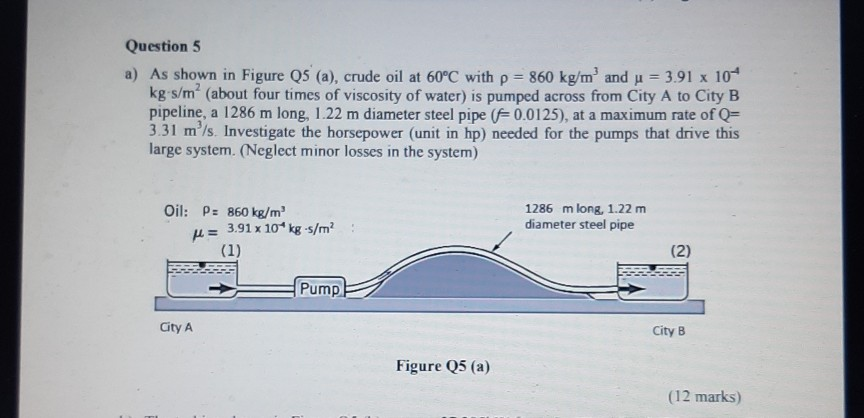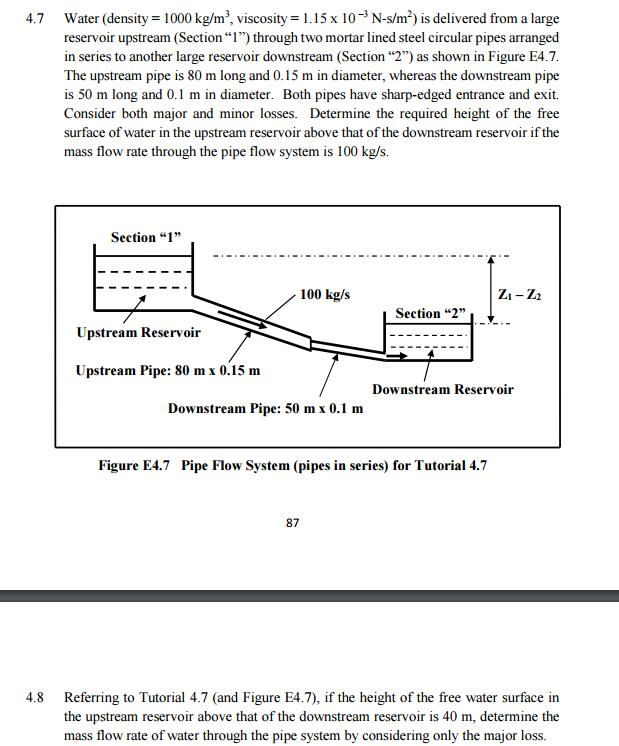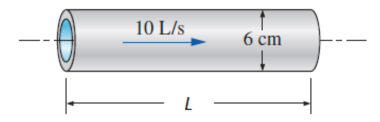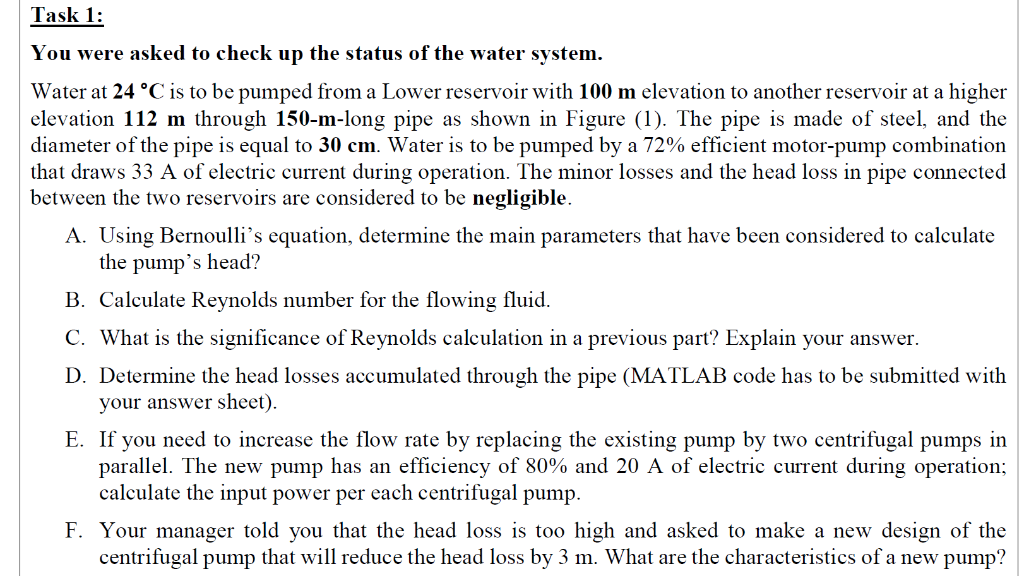QuestionRe =* v * D /= 860 * 2.83 * 1.22 / ( 3.91 * 10^-4)

= 7.6 * 10^6

so turbulent flow

first we need to calculate the head loss by Darcy's equation

head = h = f * L * (Q/A)^2 / ( 2*g*D)

area = (/4)*D^2

= (/4)*1.22^2

= 1.17 m2

Q/A = 3.31 / 1.17 = 2.83 m/s

h = 0.0125 * 1286 * 2.83^2 / ( 2*9.81*1.22)

= 5.38 m

Power = q*h*g*q(m3 /h) = Q*3600

= 3.31 * 3600

= 11916 m3/h

Power = P = 11916 * 5.38 * 9.81 * 860

= 540854227.7 W

= 725005.6672 HP

#### Earn Coins

Coins can be redeemed for fabulous gifts.

Similar Homework Help Questions
• ### Water (density = 1000 kg/m3, viscosity = 1.15 x 10-3 N-s/m2) is delivered from a large reservoir ...Water (density = 1000 kg/m3, viscosity = 1.15 x 10-3 N-s/m2) is delivered from a large reservoir upstream (Section"1") through two mortar lined steel circular pipes arranged in series to another large reservoir downstream (Section "2") as shown in Figure E4.7 The upstream pipe is 80 m long and 0.15 m in diameter, whereas the downstream pipe is 50 m long and 0.1 m in diameter. Both pipes have sharp-edged entrance and exit. Consider both major and minor losses. Determine...

• ### Water at 15°C (ρ  = 999.1 kg/m3 and μ = 1.138 × 10−3 kg/m·s) is flowing steadily in a 34-m-long and 6-cm-diameter horizontal pipe made of stainless steel at a rate of 10 L/s. Determine the pressure d...Water at 15°C (ρ  = 999.1 kg/m3and μ = 1.138 × 10−3 kg/m·s) is flowing steadily in a 34-m-long and 6-cm-diameter horizontal pipe made of stainless steel at a rate of 10 L/s. Determine the pressure drop, the head loss, and the pumping power requirement to overcome this pressure drop. The roughness of stainless steel is 0.002 mm.Determine the following:A)The pressure drop in _______  kPa.B)The head loss in _______  m.C)The pumping power requirement in _______  kW.

• ### you can skip task 1 (3) because its a code in matlab. Task 1: You were asked to check up the status of the water system. Water at 24 °C is to be pumped from a Lower reservoir with 100 m elevation to...you can skip task 1 (3) because its a code in matlab. Task 1: You were asked to check up the status of the water system. Water at 24 °C is to be pumped from a Lower reservoir with 100 m elevation to another reservoir at a higher elevation 112 m through 150-m-long pipe as shown in Figure (1). The pipe is made of steel, and the diameter ofthe pipe is equal to 30 cm water is to be pumped...